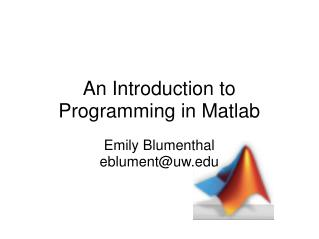DownloadDownload PresentationAn Introduction to Programming in Matlab

# An Introduction to Programming in Matlab

Download Presentation## An Introduction to Programming in Matlab

- - - - - - - - - - - - - - - - - - - - - - - - - - - E N D - - - - - - - - - - - - - - - - - - - - - - - - - - -
##### Presentation Transcript

1. An Introduction to Programming in Matlab Emily Blumenthal eblument@uw.edu

2. What is Matlab? MATrix LABoratory Stores information as N x M x … dimensional matrices Applications include Matrix manipulation Digital signal processing 3D Graphics Toolboxes for specific needs Statistics Toolbox Psychtoolbox

3. Matlab Resources Matlab tutorials: http://www.mathworks.com/academia/student_ center/tutorials/mltutorial_launchpad.html# Matlab lessons from Ione Fine and Geoff Boynton: http://courses.washington.edu/matlab1/lessons.html http://www.duke.edu/~hpgavin/matlab.html demo help COMMAND doc COMMAND helpdesk

4. The Matlab Environment List of stored variables Command history Lists commands and answers

5. Matlab as a Calculator Each result is saved in a separate variable All variables are arrays -- the fundamental data type in Matlab is a matrix; even scalars are saved as 1X1 arrays. Variables come in different sizes e.g. m X n X p X ... There are different classes of data types, default numerical data type is a double. The double data type is a floating point number with 16 digits of precision.

6. Variables in Memory Who: Gives you a list of all of the variables you have in memory Whos: Gives you the name of each variable, how long it is, how much computer memory the list uses, and the type of variable (e.g. a list of characters -- char -- or double. Disp: Displays a particular variable Clear x: deletes a particular variable from memory Clear all: deletes all variables from memory

7. M-Files and Paths File -> New -> Blank M-File Create a comment % Set a path: Path, pwd, cd

8. Saving & Reading Data .mat format save filenamevariables load filename Excel xlswrite(File, Array, Sheet, Range) xlsread(File, Sheet, Range) Can also read/write from a text file

9. Basic Plotting gcf -- get current figure (handle/pointer) gca -- get handle to current axis Change figure properties using the set command set(gcf, ‘COMMAND’, PROPERTY)

10. Basic Plotting

11. More Plotting Error bar plots: errorbar(X,Y,L,U) Plots the graph of vector X vs. vector Y with error bars specified by vectors L and U Bar Graphs: bar(X,Y) Plots a bar graph of X and Y. bar(X,Y,WIDTH) specifies the width of the bars. WIDTH>1 produces overlapping bars Histograms: hist(Y,M) Groups data Y into M bins

12. Saving Figures saveas(‘x’, FILENAME, FORMAT) E.g. saveas(gcf, ‘myhistogram’, ‘jpg’) Writes current figure to a jpg file, saved in current working directory

13. 3D Plotting mesh(X,Y,Z): plots the colored parametric mesh defined by the matrices X, Y, and Z. The axes are determined by the range of X, Y, and Z surf(X,Y,Z): plots a 3D colored surface defined by matrices X, Y, and Z help('graph3d')

14. Basic Graphics image(C): displays the current matrix as an image imagesc(C): scale data and display as an image; scaled to use full colormap colormap(MAP): sets the current figure’s color to MAP

15. Strings and Vectors String: a sequence of letters stored as a variable string1='hello world!'; Single quotes identify a string Vector: a 1 X n array of numbers vector1=[0 1 2 3 3 5 10]; Square brackets identify a vector linspace and the : operator

16. Indexing and Subscripting string1=‘hello world!’ string1(2)=e string1(12)=‘?’ >> string1(12)='?' string1 = hello world? vector1=1:10:100 >> vector1(3)=21 >> vector1(3:5)=21 31 41

17. Matrices 2 dimensional matrices: mat1=[1 2 3 4; 5 6 7 8; 9 10 11 12] mat1=[1 2 3 4 5 6 7 8 9 10 11 12] use square brackets to concatenate, enter values sequentially in rows; semicolon (;)marks the start of a new row. Column separator is a comma or a space, row separator is a semicolon or enter Zeros, ones, rand, magic, eye etc.

18. Indexing into Matrices Vectors and scalars are initialized the same way as matrices. It is important to note that MATLAB indexes matrices in the following manner: (1,1) (1,2) (1,3) (1,4) (2,1) (2,2) (2,3) (2,4) This means that the first element always has index (1,1), not (0,0).

19. Matrix Operations size(mat) Transpose using a single quote (‘) Addition and subtraction Operations with scalars Operations with vectors of the same size Scalar multiplication and division Matrix multiplication and division

20. Matrix Operations (from Matlab’s tutorial)